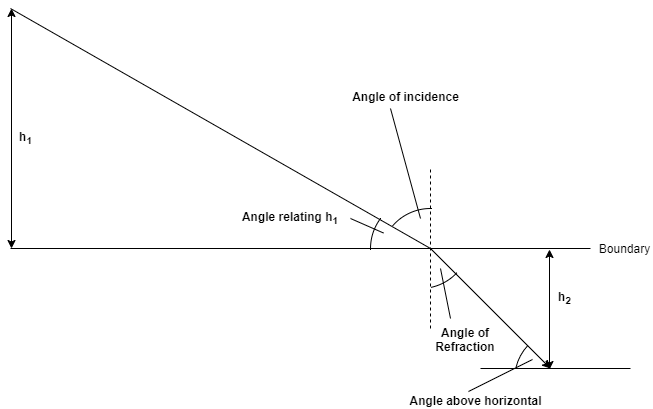# Problem: An underwater diver sees the sun 59 degrees above horizontal.How high is the sun above the horizon to a fisherman in a boat above the diver?Express your answer using two significant figures.φ= _____________degrees

###### FREE Expert SolutionThe angle above horizontal = 59°

We'll define the angle of incidence as the angle at which the ray from the sun strikes the interface.

The boat is at the boundary. Therefore, the target is angle relating h1.

The angle of refraction = 90 - 59 = 31°

Use Snell's Law to relate the Angle of Incidence with the Angle of Refraction:

84% (29 ratings)###### Problem Details

An underwater diver sees the sun 59 degrees above horizontal.

How high is the sun above the horizon to a fisherman in a boat above the diver?

Express your answer using two significant figures.

φ= _____________degrees

Frequently Asked Questions

What scientific concept do you need to know in order to solve this problem?

Our tutors have indicated that to solve this problem you will need to apply the Refraction Of Light concept. You can view video lessons to learn Refraction Of Light. Or if you need more Refraction Of Light practice, you can also practice Refraction Of Light practice problems.